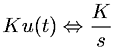Equations > Signal Processing > Laplace Transform Pairs > Laplace transform of unit step function times a constant (K)

### Laplace transform of unit step function times a constant (K)Latex Code:

MathML Code:

 $\mathrm{Ku}\left(t\right)⇔\fracKs$

MathType 5.0: